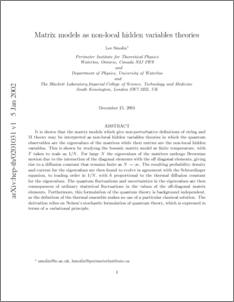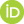# Matrix models as non-local hidden variables theories

Smolin, Lee (2001) Matrix models as non-local hidden variables theories. [Preprint]Preview
PDF
me-hidden.pdf

## Abstract

It is shown that the matrix models which give non-perturbative definitions of string and M theory may be interpreted as non-local hidden variables theories in which the quantum observables are the eigenvalues of the matrices while their entries are the non-local hidden variables. This is shown by studying the bosonic matrix model at finite temperature, with T taken to scale as 1/N, with N the rank of the matrices. For large N the eigenvalues of the matrices undergo Brownian motion due to the interaction of the diagonal elements with the off diagonal elements, giving rise to a diffusion constant that remains finite as N goes to infinity. The resulting probability density and current for the eigenvalues are then found to evolve in agreement with the Schroedinger equation, to leading order in 1/N, with hbar proportional to the thermal diffusion constant for the eigenvalues. The quantum uctuations and uncertainties in the eigenvalues are then consequences of ordinary statistical uctuations in the values of the off-diagonal matrix elements. Furthermore, this formulation of the quantum theory is background independent, as the definition of the thermal ensemble makes no use of a particular classical solution. The derivation relies on Nelson's stochastic formulation of quantum theory, which is expressed in terms of a variational principle.

 Export/Citation: EndNote | BibTeX | Dublin Core | ASCII/Text Citation (Chicago) | HTML Citation | OpenURL
 Social Networking:

Item Type: Preprint
Creators:
CreatorsEmailORCIDSmolin, Lee
Keywords: hidden variables theory, quantum gravity, foundations of quantum theory, string theory
Subjects: Specific Sciences > Physics > Quantum Field Theory
Specific Sciences > Physics > Quantum Mechanics
Specific Sciences > Physics > Relativity Theory
Depositing User: Lee Smolin
Date Deposited: 12 Jan 2002
Item ID: 528
Subjects: Specific Sciences > Physics > Quantum Field Theory
Specific Sciences > Physics > Quantum Mechanics
Specific Sciences > Physics > Relativity Theory
Date: December 2001
URI: http://philsci-archive.pitt.edu/id/eprint/528

### Monthly Views for the past 3 yearsView Item